Function Repository Resource:

# FlatManifoldToGraph

Produce random spatial graphs by sprinkling points into a flat (Euclidean) Riemannian manifold

Contributed by: Jonathan Gorard
 ResourceFunction["FlatManifoldToGraph"][dim,n] produces a random sprinkling of n points into a flat (Euclidean) Riemannian manifold of dimension dim, assuming a discreteness scale of 1. ResourceFunction["FlatManifoldToGraph"][dim,disc,n] produces a random sprinkling of n points with discreteness scale disc into a flat (Euclidean) Riemannian manifold of dimension dim. ResourceFunction["FlatManifoldToGraph"][…,"prop"] gives the property "prop" for the specified flat (Euclidean) Riemannian manifold sprinkling.

## Details and Options

When no "prop" argument is given, ResourceFunction["FlatManifoldToGraph"] returns a ManifoldGraph object.
By default, the discreteness scale is assumed to be equal to 1.
Sprinklings are performed using a Poisson point process, with the expected number of sprinkled points being proportional to the Riemannian volume measure.
Spatial edges are added in accordance with both the Riemannian metric and the specified discreteness scale, so as to guarantee approximate isometry between the spatial graph and the underlying manifold.
In ResourceFunction["FlatManifoldToGraph"][,"prop"], the following properties can be requested. These are the same as the properties supported by ManifoldGraph[]["prop"]:
 "SpatialGraph" spatial graph with vertex coordinates given by the underlying manifold coordinates "Points" plot of the sprinkled points only (without spatial edges) "PointsList" list of the manifold coordinates of all sprinkled points "DiscretenessScale" discreteness scale of the sprinkling "PointsCount" number of sprinkled points "Dimensions" number of dimensions in the continuum manifold approximation "EdgeCount" total number of spatial edges "PureSpatialGraph" spatial graph with vertex coordinate information removed "Properties" list of properties
The discreteness scale determines at what distance (with respect to the Riemannian metric) a pair of points should be regarded as adjacent.
In 3 dimensions and below, the spatial graph layout uses vertex coordinate information by default. In higher dimensions, the spring electrical embedding method is used by default.

## Examples

### Basic Examples (3)

Produce a random sprinkling of 100 points into a 2-dimensional flat (Euclidean) manifold, with discreteness scale 0.3:

 In:=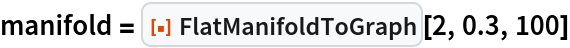Out=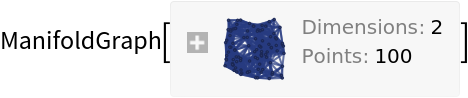Show the spatial graph:

 In:=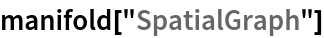Out=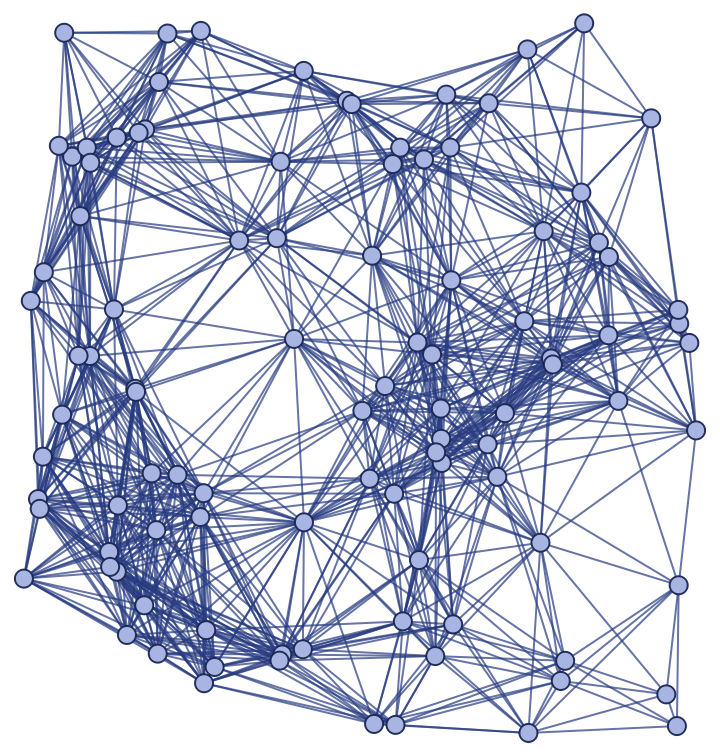Show the pure spatial graph (with vertex coordinate information removed):

 In:=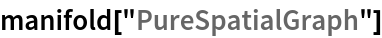Out=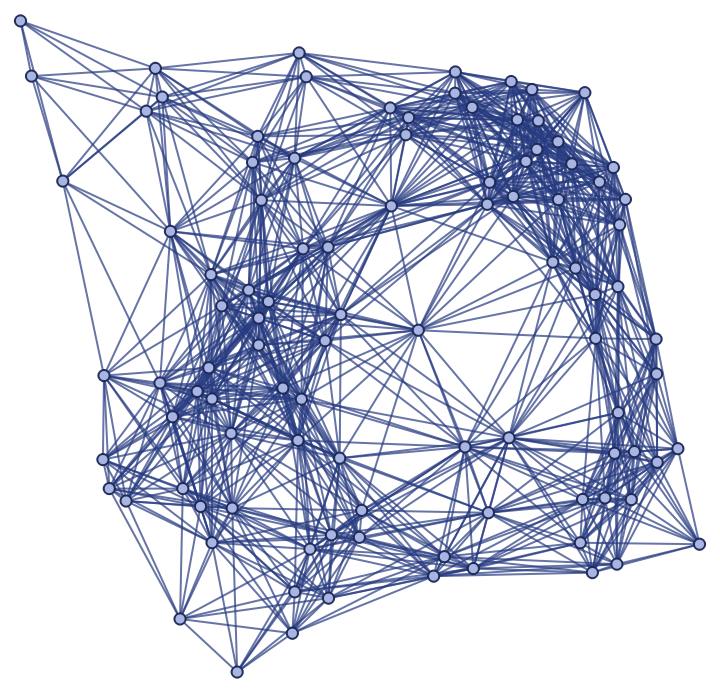Produce a random sprinkling of 200 points into a 3-dimensional flat (Euclidean) manifold, with discreteness scale 0.3:

 In:=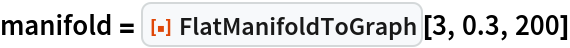Out=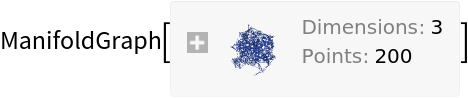Show the spatial graph:

 In:=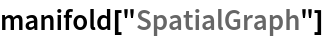Out=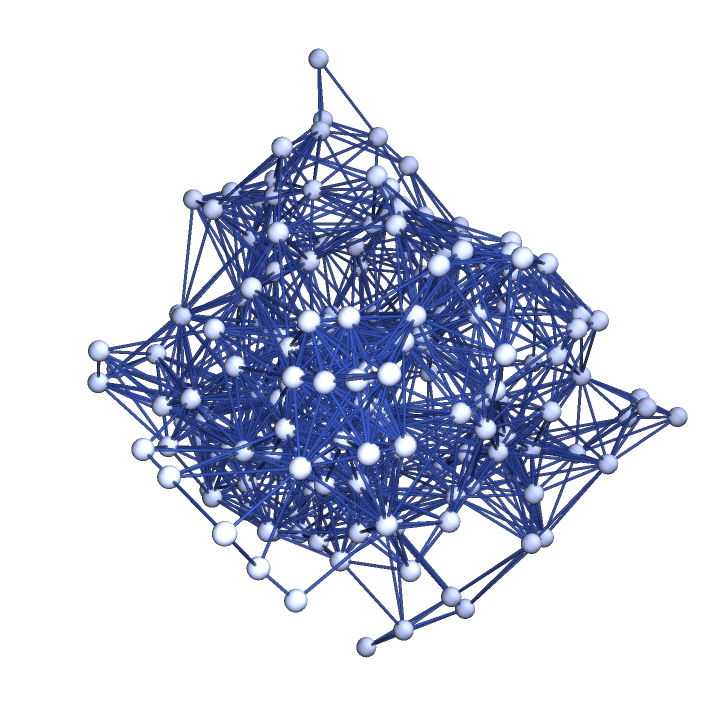Show the pure spatial graph (with vertex coordinate information removed):

 In:=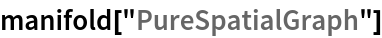Out=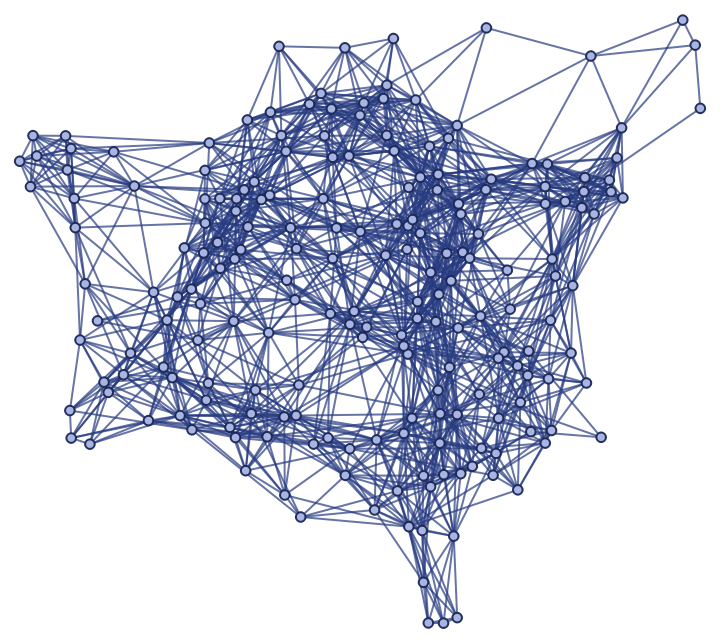Plot the positions of the sprinkled points only (without spatial edges):

 In:=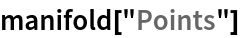Out=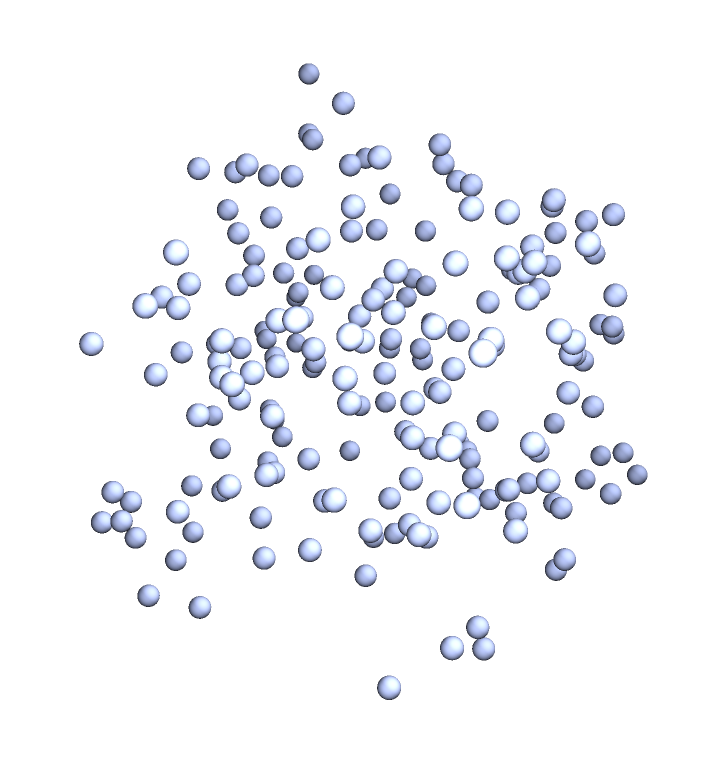By default, the discreteness scale is assumed to be equal to 1:

 In:=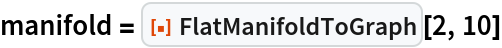Out=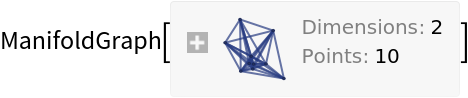Show the spatial graph:

 In:=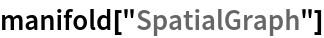Out=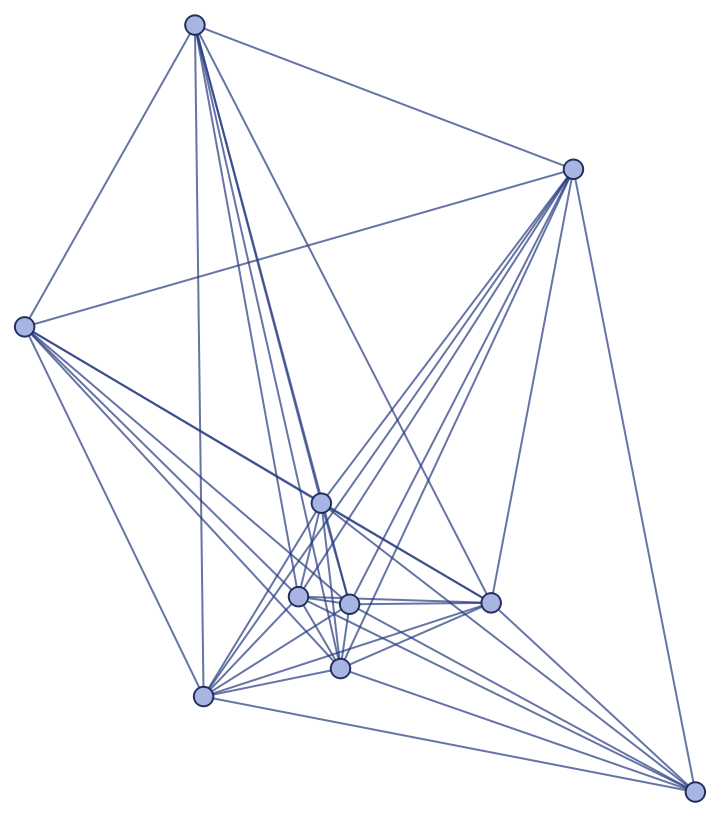### Scope (3)

Sprinklings can be performed in arbitrary (integer) numbers of dimensions:

 In:=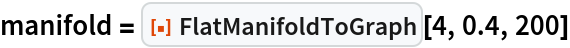Out=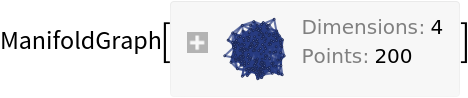In:=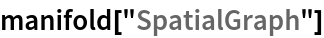Out=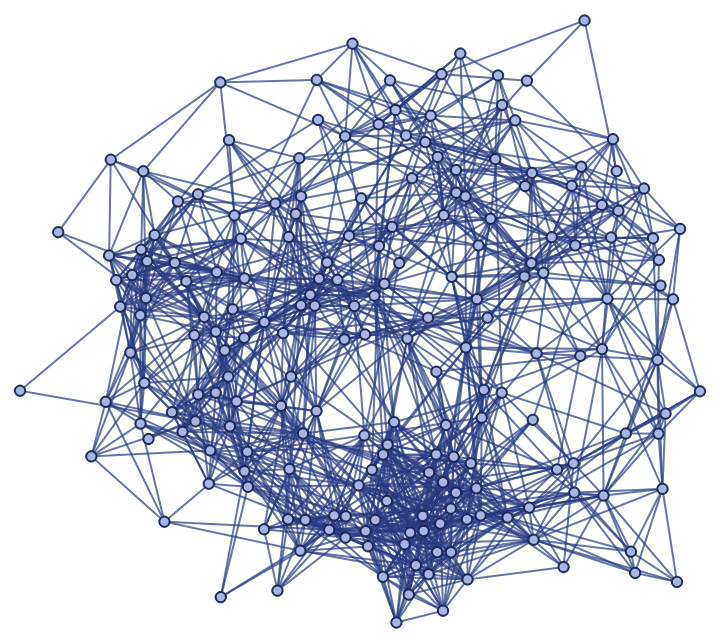In seven dimensions:

 In:=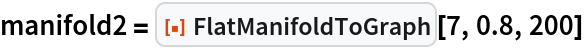Out=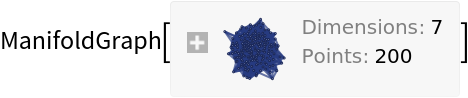In:=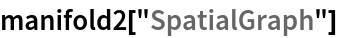Out=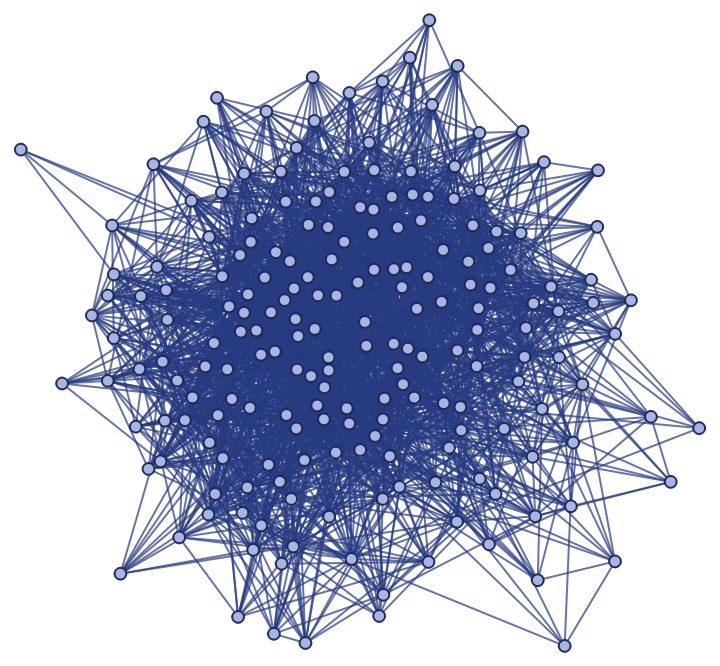Properties can be requested directly from FlatManifoldToGraph:

 In:=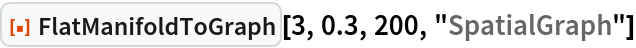Out=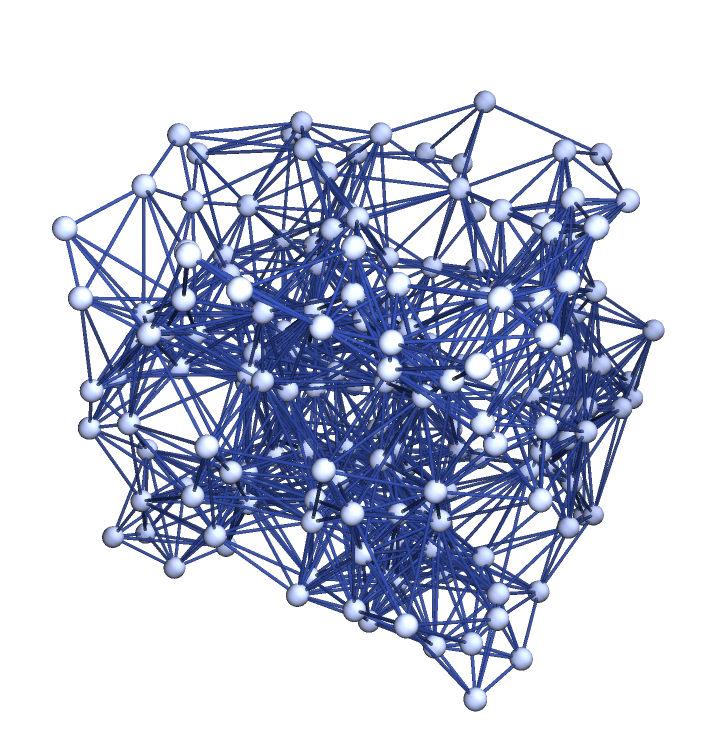Produce a random sprinkling of 200 points into a 2-dimensional flat (Euclidean) manifold, with discreteness scale 0.2:

 In:=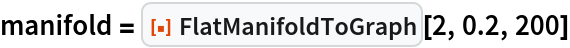Out=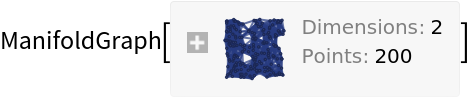Show the complete list of properties:

 In:=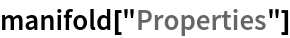Out=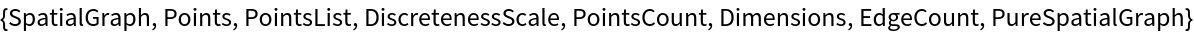Show the spatial graph:

 In:=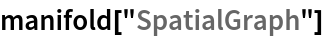Out=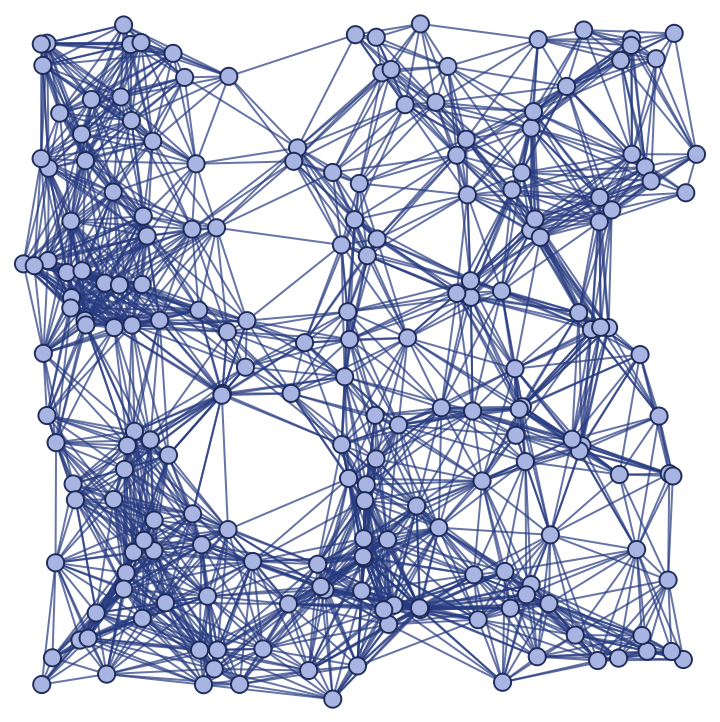Plot the positions of the sprinkled points only (without spatial edges):

 In:=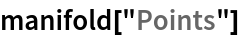Out=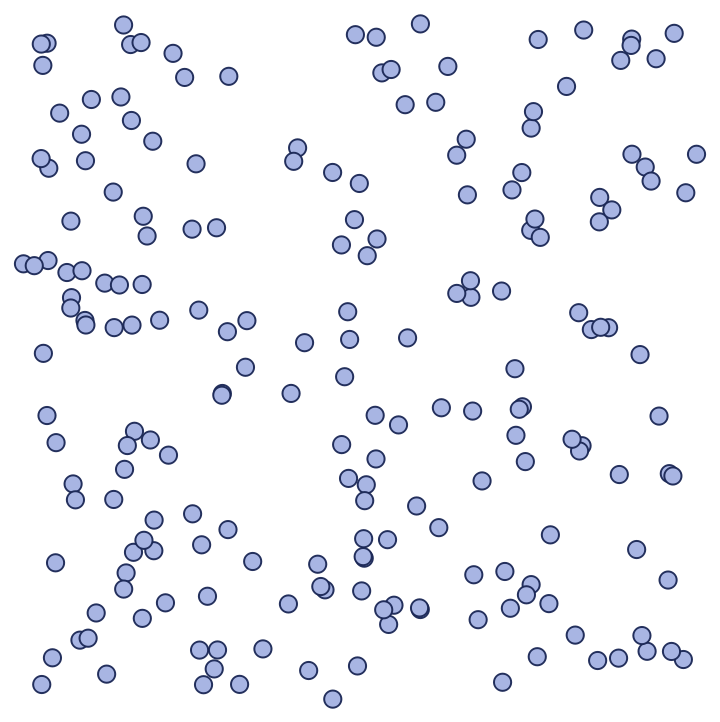Show a complete list of manifold coordinates for the sprinkled points:

 In:=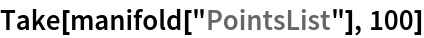Out=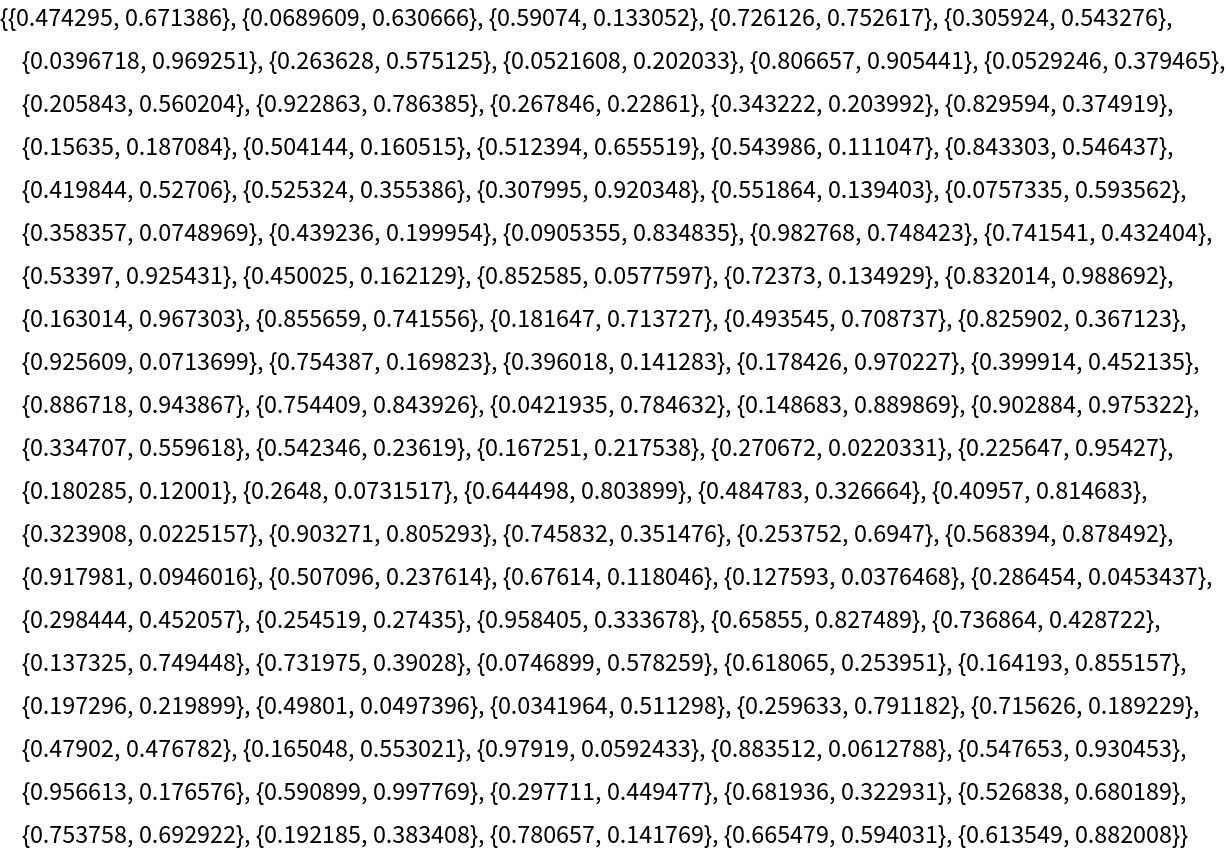Show the discreteness scale:

 In:=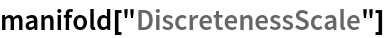Out=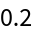Show the number of dimensions in the background manifold:

 In:=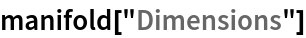Out=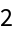Show the total number of spatial edges:

 In:=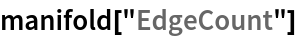Out=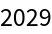Show the pure spatial graph (with vertex coordinate information removed):

 In:=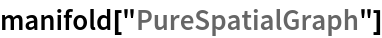Out=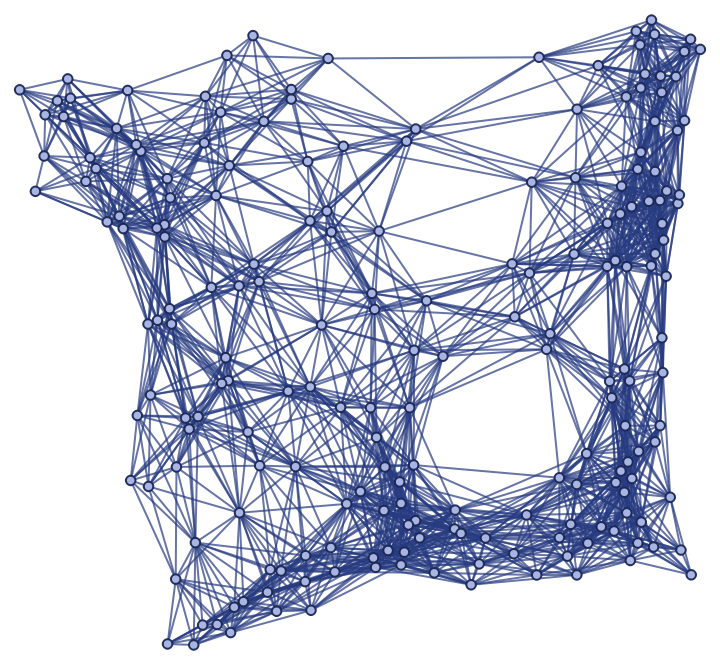Jonathan Gorard

## Version History

• 1.0.0 – 11 December 2020